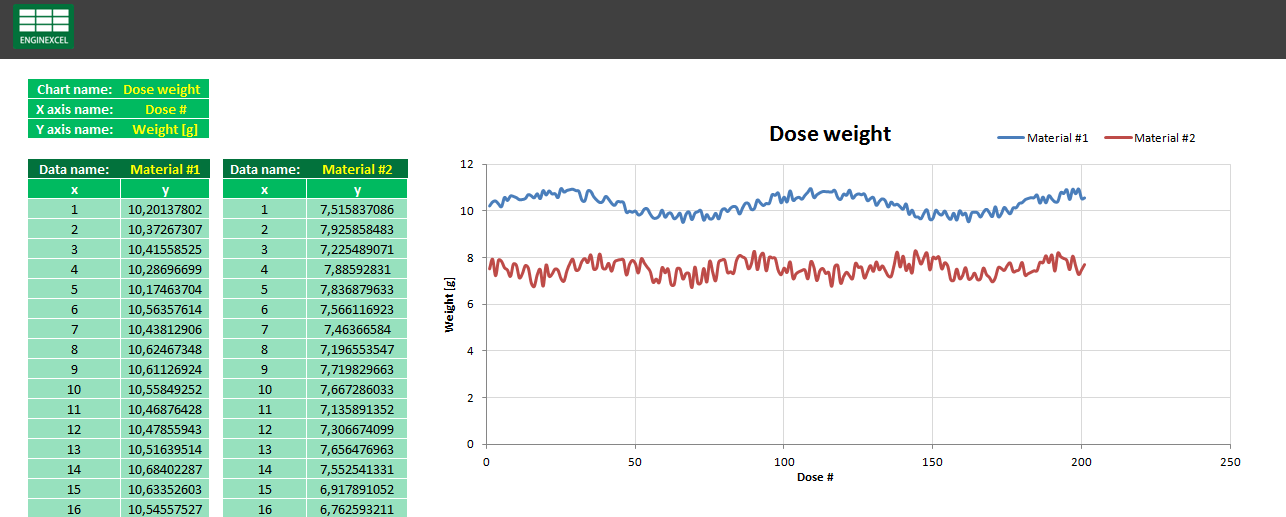# Excel Data Import And Analysis

## EnginExcel builds bespoke spreadsheets to import lab instruments and production machinery data in Excel and automatically analyse it.

Mechanical Engineers are normally quite familiar with doing and analysing tests. There are loads of testing machines that have been created during the years to provide useful data about material properties, acceleration status, weight, temperature, etc.

One of the first experiences I had with handling this type of data was at the university, where I did a tensile test to verify the properties of a material. The machine provided me an output of 4000 points, each one including one value for time, one for travel and one for force. I analysed this data with Excel, which helped me to get the results I needed.

One thing that I noticed was that Excel's calculation time gets longer and noticeable (normally it's so quick that you even don't notice it) when there is a considerable amount of data to compute. There are several techniques that can be used to reduce the time needed to refresh the page. One of the most powerful is the use of array formulas, which are known as one of the most efficient ways of handling big amounts of data in Excel (for more info about arrays formulas see this page).

During my career as an Engineer I several times appreciated how easy is to import and handle data in Excel. In one click it is possible to get the reading from a testing setup, and with a second click to visualise the results in a chart. After learning the capabilities of VBA I also started to extend Excel's possibilities. As an example, I created data import setups where it was possible to import data from various experiments together, store it in different pages, analyse it and output a result. This becomes extremely useful and saves an incredible amount of time where there is the need of analysing various experiments at the same time.The use of Excel and VBA together can also make your life easier if you have the need of doing some analysis on data while doing an experiment. Some time ago I had to do a dosing test, where I dosed some powder in a container for several times in a row. I had to try to keep the dosed amount of powder constant by adjusting some parameters, so each time I had to measure the tare of the container, measure the overall weight and then copy the values in a spreadsheet, which would tell me the trend my weights were having and any correction that may be needed. After discovering that the balance allowed the possibility of printing data to a computer, I set VBA to automatically import the weight values to Excel and analyse them. This allowed me to avoid to copy data manually (which very often leads to some typing errors) and also to fully concentrate on the experiment itself, which made it proceed more quickly and efficiently.

The application of these approaches to the Engineering world are infinite and fully customisable. These are some examples of how Excel could help with your data import and analysis:

• Import and elaboration of data from lab instrument (see example)
• Automated batch report with statistics
• Calibration procedure for liquid dosator
• Zoom and scroll of an Excel scatter plot chart (see example)

EnginExcel uses all the tools available in Excel and VBA to create custom automated spreadsheets that make the import and analysis of your data more quick and efficient.